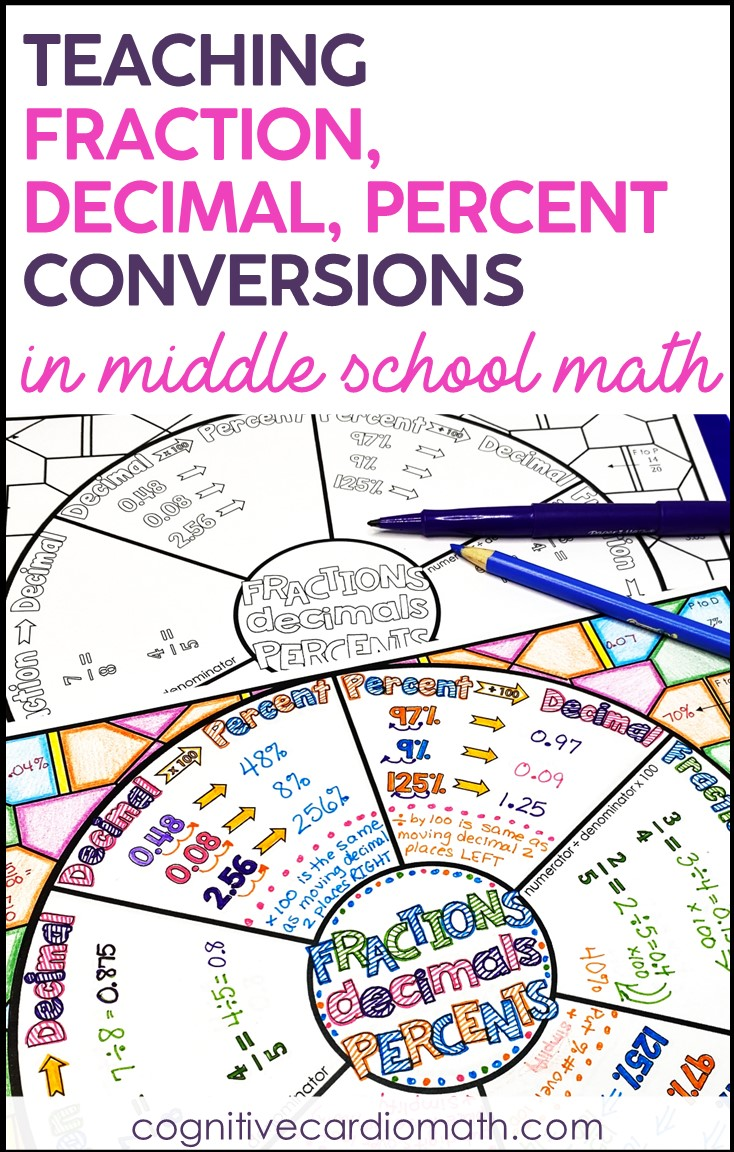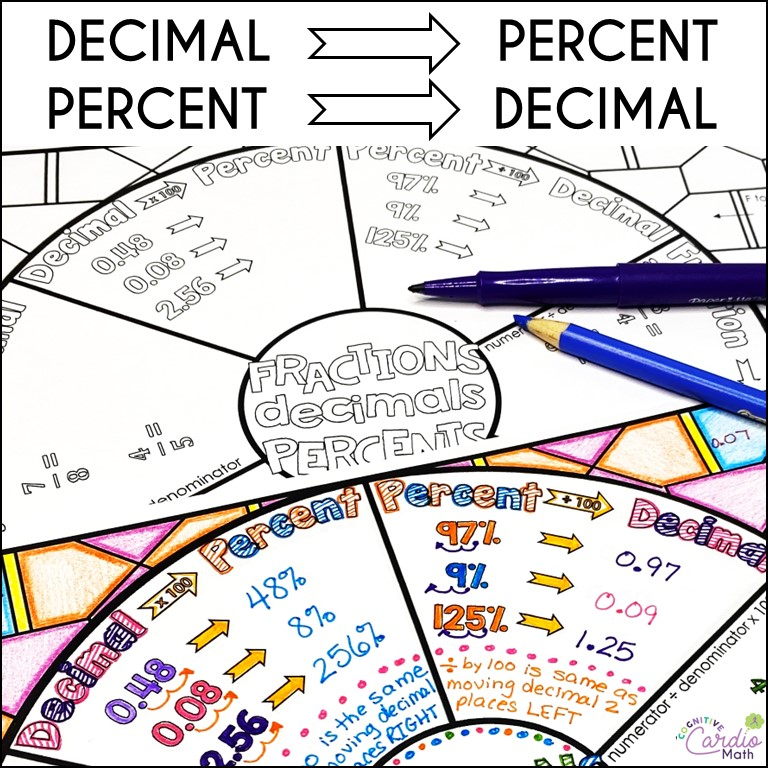# Teaching Fraction, Decimal, Percent Conversions Math Wheel

What approach do you use for  teaching fraction, decimal, percent conversions?

I’ve found that one of the best ways to teach these concepts is to use this math wheel that I’ve created.

Maybe you’re teaching fraction, decimal, percent conversions in 6th, 7th, or even 8th grade and students are still struggling with the concepts. Or, maybe some of them are pretty solid but just need some reminders.

Either way, the structure of the notes in this free math wheel can really help your students.

And, as an added bonus, the wheel can be kept in student binders and used as a reference all year!How to Use This Fraction, Decimal, Percent Conversion Math Wheel (this information is also in the free wheel download):
You can use the wheel to introduce the conversions, filling in just the ones you are covering each day.

Or, you can use it to review all the fraction, decimal, percent conversions at once. In either case, the wheel can be kept in students’ notebooks as a reference/study tool.

1) I like to begin with decimal to percent and percent to decimal

In the arrows in these sections, you’ll see x 100 and ÷ 100.

I think it’s important that students understand these are the operations being used for these conversions before giving them a ‘move the decimal point’ shortcut, so I let them use calculators to complete the examples.Once the examples are complete, I ask the students to look for a pattern, by asking what happens to the decimal point in each of these examples.

We decide on the “shortcut” rules together and then write them at the bottom of those sections.

2) The fraction to percent and fraction to decimal sections have the rules written already, so the examples just need to be completed.

• I relate the fraction to percent conversion to students grades. By the time we get to this topic during the year, students have been figuring out their grades for months (I never write their percentages on their assessments – they need to calculate
them).
• My 6th grade students know how to find their percentage if their quiz grade was 6/8 or their test was 48/52.
• However, sometimes they need a reminder that this official fraction to percent “rule” is the same thing they’ve been doing for months! I have them write a  little reminder in that section on the wheel – “just like test grades!”
3)  For percent to fraction, students need to remember that percent means “out of 100,” so the percent number will always go over 100. Then they can simplify to get to lowest terms.

4)  I find that decimal to fraction is sometimes tricky for students.

• When students have trouble, I ask them to read the decimal number according to place value (“How do you say this number, using tenths, hundredths, or thousandths, etc.?”). Once
they say the number, they know how to write the fraction. For example, 0.27 is 27 hundredths, which is 27/100.
• After completing the examples, we discuss the idea that the denominator will be whatever the last decimal place is (10, 100, 1000, etc.) and the numerator will be the digits in the decimal number. We write this rule as simply as possible.

There are practice 10 problems/numbers on the outside of the circle.

• Above each number is the conversion to complete (F to P, P to D, etc.)
• After completing the problems, students can color the wheel background.

Interested in more math wheels? Check out these free problem solving wheels!

## EllieWelcome to Cognitive Cardio Math! I’m Ellie, a wife, mom, grandma, and dog ‘mom,’ and I’ve spent just about my whole life in school! With nearly 30 years in education, I’ve taught:

• All subject areas in 4th and 5th grades
• Math, ELA, and science in 6th grade (middle school)

I’ve been creating resources for teachers since 2012 and have worked in the elearning industry for about five years as well!